# Assertion & Reason Test: Alcohols, Phenols & Ethers

## 16 Questions MCQ Test Topic-wise MCQ Tests for NEET | Assertion & Reason Test: Alcohols, Phenols & Ethers

Description
Attempt Assertion & Reason Test: Alcohols, Phenols & Ethers | 16 questions in 30 minutes | Mock test for NEET preparation | Free important questions MCQ to study Topic-wise MCQ Tests for NEET for NEET Exam | Download free PDF with solutions
QUESTION: 1

### Directions : In this question, A statement of Assertion (A) is followed by a statement of Reason (R). Mark the correct choice as. Assertion (A): (CH3)3C−O−CH3 gives (CH3)3C−I and CH3OH on treatment with HI. Reason (R): The reaction occurs by SN1 mechanism.

Solution:

(CH3)3C−O−CH3 gives (CH3)3C−I and CH3OH on treatment with HI. The reaction occurs by SN1 mechanism.

QUESTION: 2

### Directions : In this question, A statement of Assertion (A) is followed by a statement of Reason (R). Mark the correct choice as. Assertion (A): Ethers behave as bases in the presence of mineral acids. Reason (R) : In ethers, oxygen consists of lone pair of electrons.

Solution:

Ethers behave as bases in the presence of mineral acids like HCl due to presence of lone pair of electrons on oxygen atom and form stable oxonium salts with mineral acids.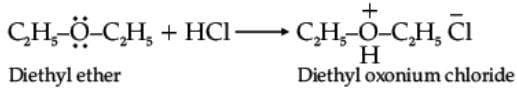QUESTION: 3

### Directions : In this question, A statement of Assertion (A) is followed by a statement of Reason (R). Mark the correct choice as. Assertion (A): Methoxy ethane reacts with HI to give ethanol and iodomethane. Reason (R): Reaction of ether with HI follows SN2 mechanism.

Solution:

Methoxy ethane reacts with HI to give ethanol and iodomethane. Reaction of ether with HI follows SN2 mechanism.

QUESTION: 4

Directions : In this question, A statement of Assertion (A) is followed by a statement of Reason (R). Mark the correct choice as.

Assertion (A): Ortho and para-nitrophenol can be separated by steam distillation.
Reason (R): Ortho isomer associates through intermolecular hydrogen bonding while para isomer associates through intermolecular hydrogen bonding.

Solution:

Ortho isomer has intermolecular H-bonding while para isomer has intermolecular hydrogen bonding.

QUESTION: 5

Directions : In this question, A statement of Assertion (A) is followed by a statement of Reason (R). Mark the correct choice as.

Assertion (A): Carboxylic acids are more acidic than phenols.
Reason (R): Phenols are ortho and para directing.

Solution:

Carboxylic acids are more acidic than phenols as the carboxylate ion, the conjugate base of carboxylic acid is stabilized by two equivalent resonance structures. Thus, the negative charge is delocalized effectively. However, in phenols, negative charge is less effectively delocalized over oxygen atom and carbon atoms in phenoxide ion.

QUESTION: 6

Directions : In this question, A statement of Assertion (A) is followed by a statement of Reason (R). Mark the correct choice as.

Assertion (A) : The C-O-C bond angle in ethers is slightly less than tetrahedral angle.
Reason (R): Due to the repulsive interaction between the two alkyl groups in ethers.

Solution:

The C-O-C bond angle in ether is slightly greater than tetrahedral angle. It is due  to the repulsive interaction between the two alkyl groups in ethers.

QUESTION: 7

Directions : In this question, A statement of Assertion (A) is followed by a statement of Reason (R). Mark the correct choice as.

Assertion : In Lucas test, 3º alcohols react immediately.
Reason : An equimolar mixture of anhyd. ZnCl2 and conc. HCl is called Lucas reagent.

Solution:
QUESTION: 8

Directions : In this question, A statement of Assertion (A) is followed by a statement of Reason (R). Mark the correct choice as.

Assertion : Phenol is more reactive than benzene towards electrophilic substitution reaction.
Reason : In the case of phenol, the intermediate carbocation is more resonance stabilized.

Solution:

-OH group shows + M effect and is an activating group, moreover the arenium ion of phenolic substitution is more stable.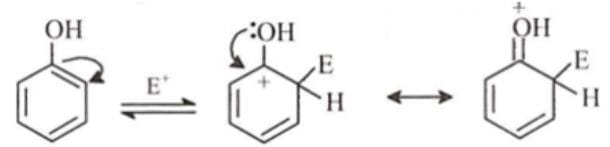QUESTION: 9

Directions : In this question, A statement of Assertion (A) is followed by a statement of Reason (R). Mark the correct choice as.

Assertion : ter – Butyl methyl ether is not prepared by the reaction of ter-butyl bromide with sodium methoxide.
Reason : Sodium methoxide is a strong nucleophile.

Solution:

In Williamson's synthesis, if a tertiary alkyl halide is used, an alkene is the only reaction product and no ether is formed.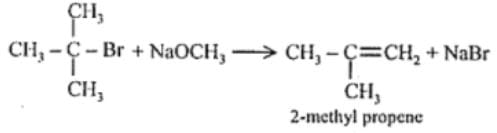It is because alkoxides are not only nucleophiles but also strong bases as well. They react with alkyl halides leading to elimination reaction.

QUESTION: 10

Directions : In this question, A statement of Assertion (A) is followed by a statement of Reason (R). Mark the correct choice as.

Assertion : With HI, anisole gives iodobenzene and methyl alcohol.
Reason : Iodide ions combine with smaller groups to avoid steric hindrance.

Solution:
QUESTION: 11

Directions : In this question, A statement of Assertion (A) is followed by a statement of Reason (R). Mark the correct choice as.

Assertion : Ethyl phenyl ether on reaction with HBr form phenol and ethyl bromide.
Reason : Cleavage of C–O bond takes place on ethyl￾oxygen bond due to the more stable phenyl-oxygen bond.

Solution:
QUESTION: 12

Directions : In this question, A statement of Assertion (A) is followed by a statement of Reason (R). Mark the correct choice as.

Assertion : The bond angle in alcohols is slightly less than the tetrahedral angle.
Reason : In alcohols, the oxygen of –OH group is attached to sp3 hybridized carbon atom.

Solution:
QUESTION: 13

Directions: These questions consist of two statements, each printed as Assertion and Reason. While answering these questions, you are required to choose any one of the following four responses.

Assertion : Reimer-Tiemann reaction of phenol with CCl4 in NaOH at 340 K gives salicyclic acid as the major product.
Reason : The reaction occurs through intermediate formation of dichlorocarbene.

Solution:

Nucleophilic attack of phenolate ion through the ortho-carbon atom occurs on CCl4 to form an intermediate which on hydrolysis gives salicylic acid.

QUESTION: 14

Directions : In this question, A statement of Assertion (A) is followed by a statement of Reason (R). Mark the correct choice as.

Assertion : In case of phenol, bromination takes place even in absence of Lewis acid whereas bromination of benzene takes place in presence of Lewis acid like FeBr3.
Reason : – OH group attached to benzene ring is highly deactivating.

Solution:
QUESTION: 15

Directions : In this question, A statement of Assertion (A) is followed by a statement of Reason (R). Mark the correct choice as.

Assertion : Ethers behave as bases in the presence of mineral acids.
Reason : Due to the presence of lone pairs of electrons on oxygen.

Solution:

Due to the presence of lone pairs of electrons on oxygen atoms, ethers behave as base and form stable oxonium salts with mineral acids.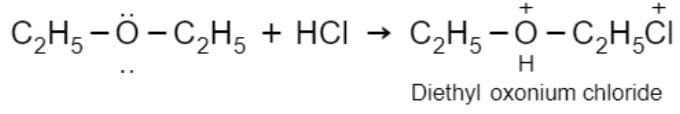QUESTION: 16

Directions : In this question, A statement of Assertion (A) is followed by a statement of Reason (R). Mark the correct choice as.

Assertion : With HI at 373 K, ter-butyl methyl ether gives ter-butyl iodide and methanol.
Reason : The reaction occurs by SN2 mechanism.

Solution:

The cleavage of C-O bond in ether takes place under drastic conditions with excess of hydrogen halides.
When one of the alkyl groups is a tertiary group, the halide formed is a tertiary halide.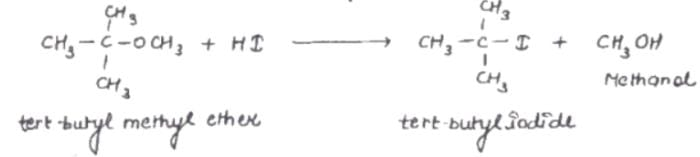Mechanism:
(i) protonation of tert-butyl methyl ether-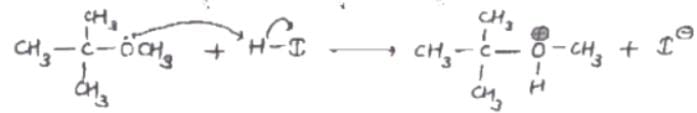(ii)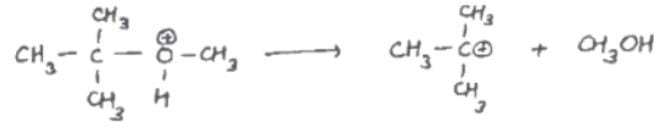In the second step, the departure of the learning group yields a tertiary i.e. a stable carbocation so the reaction proceeds through an SN’ mechanism.
(iii)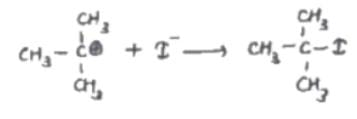So, The correct option is c.Use Code STAYHOME200 and get INR 200 additional OFF Use Coupon Code